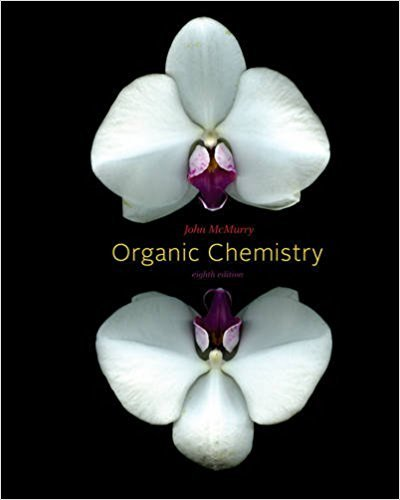×
×

# Solutions for Chapter 30.7: Sigmatropic Rearrangements## Full solutions for Organic Chemistry | 8th Edition

ISBN: 9780840054449Solutions for Chapter 30.7: Sigmatropic Rearrangements

Solutions for Chapter 30.7
4 5 0 376 Reviews
30
4
##### ISBN: 9780840054449

Chapter 30.7: Sigmatropic Rearrangements includes 1 full step-by-step solutions. Organic Chemistry was written by and is associated to the ISBN: 9780840054449. This textbook survival guide was created for the textbook: Organic Chemistry, edition: 8. Since 1 problems in chapter 30.7: Sigmatropic Rearrangements have been answered, more than 43395 students have viewed full step-by-step solutions from this chapter. This expansive textbook survival guide covers the following chapters and their solutions.

Key Business Terms and definitions covered in this textbook

the tendency for the mix of unobserved attributes to become undesirable from the standpoint of an uninformed party

• Arrow’s impossibility theorem

a mathematical result showing that, under certain assumed conditions, there is no scheme for aggregating individual preferences into a valid set of social preferences

• budget deficit

an excess of government spending over government receipts

• closed economy

an economy that does not interact with other economies in the world

• Coase theorem

the proposition that if private parties can bargain without cost over the allocation of resources, they can solve the problem of externalities on their own

• complements

two goods for which an increase in the price of one leads to a decrease in the demand for the other

• economic profit

total revenue minus total cost, including both explicit and implicit costs

• equilibrium

a situation in which the market price has reached the level at which quantity supplied equals quantity demanded

• imports

goods produced abroad and sold domestically

• income effect

the change in consumption that results when a price change moves the consumer to a higher or lower indifference curve

• marginal cost

the increase in total cost that arises from an extra unit of production

• median voter theorem

a mathematical result showing that if voters are choosing a point along a line and each voter wants the point closest to his most preferred point, then majority rule will pick the most preferred point of the median voter

• natural monopoly

a monopoly that arises because a single firm can supply a good or service to an entire market at a smaller cost than could two or more firms

• nominal interest rate

the interest rate as usually reported without a correction for the effects of inflation

• producer price index

a measure of the cost of a basket of goods and services bought by firms

• reserve ratio

the fraction of deposits that banks hold as reserves

• reserve requirements

regulations on the minimum amount of reserves that banks must hold against deposits

• total cost

the market value of the inputs a firm uses in production

• total revenue (in a market)

the amount paid by buyers and received by sellers of a good, computed as the price of the good times the quantity sold

• variable costs

costs that vary with the quantity of output produced

×